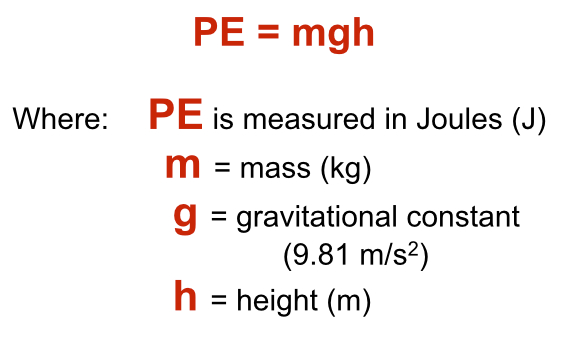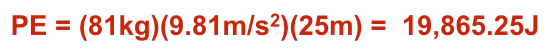# Concept Day### SLIDE KINETIC-1-1

Note: In this Investigation, we wish to develop a firm understanding of the Law of Conservation of Energy and to establish the meaning of both potential and kinetic energy.

• The Law of Conservation of Energy states that energy can neither be created nor destroyed, however it can change forms.
• Looking at the big picture, this and the Law of Conservation of Matter (that matter can neither be created nor destroyed) are extremely significant.
• It means that all energy and matter that exists in the Universe came into existence at the moment of the Big Bang, almost 14 billion years ago, and will never change. Never!

Note: On a more practical level, the Law of Conservation of Energy, like the Law of Conservation of Matter, helps us understand much more concrete phenomena that we encounter every day.

______________________________________________### SLIDE KINETIC-1-2

Note: We need to establish early on that kinetic energy is the energy of movement.

• Movement is easy to visualize when a race car speeds by or an explosion occurs. However, many times kinetic energy refers to the motion of molecules that we cannot see.
• When the molecules of an object move more rapidly, they too have greater kinetic energy. We can detect this molecular movement as heat. So heat is a form of kinetic energy.

Note: Another two examples of kinetic energy that might not be obvious to you are electrical and light energy. Both of these forms of energy are kinetic energy because of movement. In the case of electricity, it is the movement of electrons through a wire or a bolt of lightning through the atmosphere. In the case of light, it is the movement of very small particles called photons.

Note: On the left of this slide are images and words that should help you get the “feel” that kinetic energy implies movement, action, heat, light, and so on.

______________________

• Potential energy is the energy that is stored in an object or substance.

Note: This is probably a bit more abstract and harder for you to visualize than the concept of kinetic energy. Whereas it is easy to visualize the kinetic energy (the energy of movement) of a falling ball, the potential energy of the ball before it falls is much harder to visualize. It is our job in this Investigation to help you see and appreciate potential energy and its interplay with kinetic energy.

• Some examples of potential energy are shown on the right side of this slide.
• The runner is at the starting block, ready to go. He has stored chemical potential energy in his muscles which will be converted into kinetic energy when the gun sounds.
• In a similar manner, gasoline contains stored chemical potential energy that will be released as heat, sound, and mechanical kinetic energy.
• The battery stores electrons that will begin moving (become kinetic) the moment it is inserted into a complete circuit.
• Finally, the archer in the back has drawn his bow. At this point, all of the energy in the bow and arrow system is in a potential form, stored in the fibers of the bent bow. Upon release, the bow will spring back to its original shape and all of its stored potential energy will be transferred to the arrow, and kinetic energy will be propel it toward the target.

Note: We will discuss the potential/kinetic energy conversion of the drawn bow in greater detail in slide KINETIC-1-6 below.

______________________________________________### SLIDE KINETIC-1-3

Note: Investigation 1 is mainly concerned with Gravitational Potential and Kinetic Energy. This is a good place to start, as you are probably most familiar with this type of potential energy. It is the energy associated with falling objects, so let’s use an exciting example, cliff diving. The basic formula for potential energy (PE) here is:• In this example, the diver’s mass is 81 kg and the height is 25 meters. The gravitational constant is always (on Earth, at least) 9.81 m/s2.

Note: A unit of time referred to as a second squared (s2) may be an odd concept for you. A square meter (m2) or even a cubic meter (m3) makes sense, but a time squared may prompt questions. The reason for the exponent is because the gravitational constant is an acceleration, which means it is a change in velocity over time. Since velocity, measured in meters per second (m/s), already accounts for time, a change in velocity with time (acceleration) introduces a second multiplier of time, and therefore the exponent (m/s2). Don’t think of m/s2 as meters per second squared. Think of it as “meters per second per second”.

• The actual calculation for the PE of the diver is straight forward:• When small masses are used, as we will use in Lab this Investigation, we may want to use grams instead of kilograms for mass. When we do, the result will give us millijoules (mJ) rather than Joules (J).

Note: This is explained further in your Student Data Record.

______________________________________________### SLIDE KINETIC-1-4

• This slide provides practice on working through several more Gravitational Potential Energy problems.

Note: A second important aspect of this slide is that it provides us with a perfect platform to introduce the important concept of the conversion of potential to kinetic energy.

• As the diver loses potential energy during the fall, he gains kinetic energy. At the moment he hits the water, all of the initial potential energy he had before he jumped will have been converted to the kinetic energy of motion. Thus, when he hits the surface of the water, he will have 19,865.25J of kinetic energy!

______________________________________________### SLIDE KINETIC-1-5

Note: This slide is wonderful to continue the discussion of the interconversion of potential and kinetic energy.

• This slide shows various positions of a pendulum.
• Starting from the left, before the ball is released, 100% of the energy is in the form of potential energy.
• If we knew the height and mass of the ball we could easily calculate the potential energy and therefore the total energy.

Note: You will do this very thing in Lab in Investigation 1.

• When the ball is released, the potential energy decreases as it is driven by gravity toward the perpendicular. At exactly the same time, the kinetic energy of the system increases.
• Halfway down, for example, 50% of the total energy is in the form of kinetic energy and 50% is still in the form of potential energy.
• At the perpendicular, 100% of the total energy is in the form of kinetic energy, there is no more stored, potential energy.
• Unlike the situation with the cliff diver, the pendulum ball continues on. As it does, it gains height due to the push of its kinetic energy, and therefore gains potential energy.
• As it gains more and more potential energy, it loses an equal amount of kinetic energy, slows down, and stops momentarily to change directions.
• Thus, when it reaches the top of its arc to the right, all of the energy in the system is once again 100% potential and stored. There is momentarily no movement, no kinetic energy whatsoever.
• Just as when you throw a ball into the air and it momentarily pauses at its peak before falling back to Earth. After this momentary pause, the pendulum will be forced down by gravity once again and the cycle will be repeated in the opposite direction.

Note: You may think about this potential/kinetic energy interconversion in reference to the pendulum as similar to the situation when riding on a playground swing. This is absolutely accurate and a trip to the playground would certainly be instructive.

______________________________________________### SLIDE KINETIC-1-6

Note: This slide provides you with another opportunity to consider the potential/kinetic energy conversion.

• When a bow is drawn, energy is stored in the structure of the deformed bow. There is no movement; there is no kinetic energy. All of the energy in the bow is potential energy.

Note: In the example in the slide, the amount of stored potential energy is shown to be 35 J. This is a reasonable amount of energy for draw on a bow.

Note: This slide is animated so that the teacher may question students as to the amount of kinetic energy the arrow will have when released from the bowstring. The answer is 35J.

• Once the string is released and the bow snaps back to its original shape, potential energy quickly collapses to zero.
• All of the stored potential energy is immediately converted to the kinetic energy of the arrow.
• Thus, in this example, we see how energy can be transferred from one object (the bow) to another (the arrow). The Newton Cradle toy demonstrates this same principle very clearly. Observe how the moving ball transfers its kinetic energy from one ball to the next until it is transferred to the end ball on the other side.______________________________________________### SLIDE KINETIC-1-7

• The final slide simply restates the Law of Conservation of Energy but accentuates the second half of the Law; regarding the changing of one form of energy to another.
• Up to this point, and in the Lab for Investigation 1, we have been concerned with Gravitational Potential and Kinetic Energy.
• The picture in the center is a great example of this type of energy:
• The child on the slide is increasing kinetic energy while decreasing his potential energy.
• The child on the ladder, if climbing up, is increasing his potential energy, if climbing down, decreasing it.
• The children on the swings are either increasing or decreasing their potential energy depending on their direction, up or down.
• The girl under the slide isn’t using much total energy one way or another!

Note: The conversion of electrical to heat and light energy will be the subject of Investigation 2 and the conversion of chemical energy to mechanical energy will be addressed in Investigation 3.